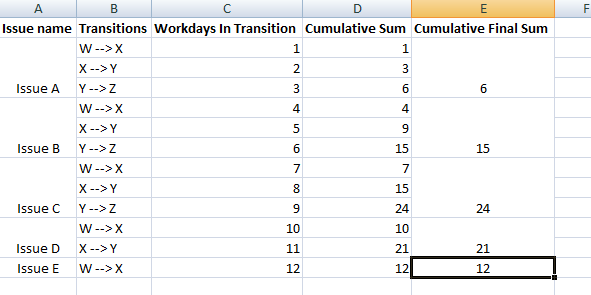# Calculate Cumulative Sum only for the rows of a Particular Issue

Hi All,

I need to find the cumulative sum of of my column - Avg Workdays in Transition for each issue.

I have a report say as follows :I have the constraints as follows:
1.Each Issue may not have all the transitions. So, the rows vary, as you can see in the picture. e.g. Issue A has 3 transitions , but Issue D can have 2, even Issue E has 1. It can happen.

Q1. How to find the cumulative sum for each issue , like mentioned in the picture.

Q2. How to find the cumulative sum and only show it in the last column for that issue, like the column Cumulative Final Sum

Regards,
Soumen

Hi,

Try creating a new calculated measure (with integer formatting) using this formula

``````CASE WHEN
[Measures].[Workdays in transition status]>0
THEN
Sum(
Order(
Filter(
[Transition].[Transition].Members,
[Measures].[Workdays in transition status]>0
),
[Transition].CurrentMember.key,
BASC
),
Rank(
[Transition].CurrentMember,
Order(
Filter(
[Transition].[Transition].Members,
[Measures].[Workdays in transition status]>0
),
[Transition].CurrentMember.key,
BASC
)
)
),
[Measures].[Workdays in transition status]
)
END
``````

Then you could create a calculated member in the “Transition” dimension using aggregate to force the correct order for Transition dimension members.

Martins / eazyBI support